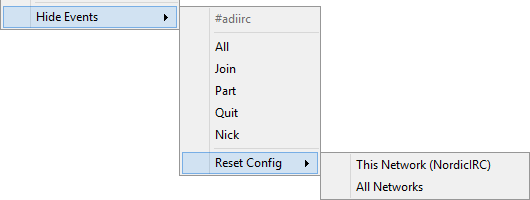[deprecated] [Script] Simple selective join/part/quit message blacklist

This script will halt (hide) the message for events Join/Part/Quit in channel/network that user enable it.

Screenshot:¶Changelog:¶

• v0.4.2
- Now it is possible to reset config per network or global.
• v0.4.1
- Added option to uncheck all events at once.
• v0.4
- New event control added: Nick change.
• v0.3
- New submenu to check all events at once.
- Fixed the toggle quit event.
• v0.2
- By request, now all config are done by channel right click menu.
• v0.1
- initial release.

Script:¶

; Simple selective join/part/quit message blacklist
; v0.4.2 by pereba

-
Hide Events
.\$style(2) #:/
.-
.\$iif(\$getVar(Join) != 1 && \$getVar(Part) != 1 && \$getVar(Quit) != 1 && \$getVar(Nick) != 1, All):\$setVar(Join) | \$setVar(Part) | \$setVar(Quit) | \$setVar(Nick)
.\$iif(\$getVar(Join) == 1 && \$getVar(Part) == 1 && \$getVar(Quit) == 1 && \$getVar(Nick) == 1, Uncheck All):\$unSetVar(Join) | \$unSetVar(Part) | \$unSetVar(Quit) | \$unSetVar(Nick)
.\$iif(\$getVar(Join) == 1, \$style(1)) Join: \$iif(\$getVar(Join) == 1, \$unSetVar(Join), \$setVar(Join))
.\$iif(\$getVar(Part) == 1, \$style(1)) Part: \$iif(\$getVar(Part) == 1, \$unSetVar(Part), \$setVar(Part))
.\$iif(\$getVar(Quit) == 1, \$style(1)) Quit: \$iif(\$getVar(Quit) == 1, \$unSetVar(Quit), \$setVar(Quit))
.\$iif(\$getVar(Nick) == 1, \$style(1)) Nick: \$iif(\$getVar(Nick) == 1, \$unSetVar(Nick), \$setVar(Nick))
.-
.Reset Config
..This Network ( \$+ \$network \$+ ):if (\$\$?!"Erase events control config for \$network network?") { unset %Hide.Events* \$+ \$network \$+ .* }
..All Networks:if (\$\$?!"Erase events control config for ALL networks/channels?") { unset %Hide.Events* }
-
}

alias -l getVar { return %Hide.Events. [ \$+ [ # ] \$+ . \$+ [ \$network ] \$+ . \$+ [ \$1 ] ] }

alias -l setVar { set %Hide.Events. \$+ # \$+ . \$+ \$network \$+ . \$+ \$1 1 }

alias -l unSetVar { unset %Hide.Events. [ \$+ [ # ] \$+ . \$+ [ \$network ] \$+ . \$+ [ \$1 ] ] }

on ^*:JOIN:*:{
if (\$getVar(Join) == 1) {
halt
}
}

on ^*:PART:*:{
if (\$getVar(Part) == 1) {
halt
}
}

on ^*:QUIT:{
var %i = 1
while (\$comchan(\$nick,%i)) {
var %x %Hide.Events. [ \$+ [ \$comchan(\$nick,%i) ] \$+ . \$+ [ \$network ] \$+ . \$+ Quit ]
if (%x != 1) {
echo 27 -trbf \$comchan(\$nick,%i) * \$chr(2) \$+ \$nick \$+ \$chr(2) ( \$+ \$address \$+ ) has quit IRC ( \$+ \$1- \$+ )
}
inc %i
}
halt
}

on ^*:NICK:{
var %i = 1
while (\$comchan(\$newnick,%i)) {
var %x %Hide.Events. [ \$+ [ \$comchan(\$newnick,%i) ] \$+ . \$+ [ \$network ] \$+ . \$+ Nick ]
if (%x != 1) {
echo 33 -trbf \$comchan(\$newnick,%i) * \$chr(2) \$+ \$nick \$+ \$chr(2) is now known as \$chr(2) \$+ \$newnick \$+ \$chr(2)
}
inc %i
}
halt
}

✔ mIRC compatible.